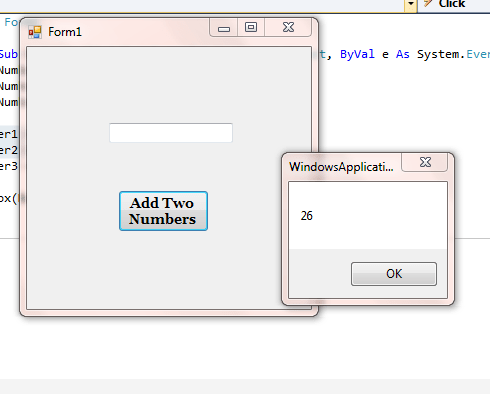VB.NET Tutorial 5: Writing Code For Simple Mathematical Calculations

In last tutorial, we have created a form with a button that says “Add Two Numbers” and a textbox. Double click on button to open coding windows and type following code into it. You can also copy paste it from here but typing will introduce you with possible errors you can make while writing own code. It will also help you remember syntax of particular function. So we suggest to type it rather than just copy pasting.

```Dim Number1 As Integer
Dim Number2 As Integer
Dim Number3 As Integer

Number3 = Number1 + Number2

MsgBox(Number3)```

Save the code and debug it by pressing F5. Something like this must be on your screen now:We already know what Dim line of code represents. We defined three such variables as Number1, Number2 and Number3. We assigned result of addition to variable Number3 with help of line

Number3= Number1 + Number2

Message box is used in this case to display result of addition. We used a very simple code for such visuals:

MsgBox (Number3)

From this code, we tell VB to display value stored in variable Number3 after calculation, in a message box that must pop up after clicking on button. And it works!

But then what is use of textbox that we have included in form? Let us use it to display our result. But for that, we need to make minor changes to out code. Delete Message box code from our lines of code and replace it with:

`Textbox1.Text = Number3`

Now debug the code and click on button. You will see something like this on screen:What we done here it very simple. We accessed Text property of “Textbox1” in our form. We used this property to display value stored in variable Number3.

This small line of code has taught you how to perform addition using a button and textbox in VB. Performing any basic mathematical calculations in VB is as simple as this. Our readers can try out coding for subtraction, multiplication and division on their own as part of practice. You won’t need individual textbox for each calculation but this one textbox can be used with three other buttons.

Try it out and let us know if you have any questions.

1.meow says:
1.trolol says: